﻿ R小姐：重复测量方差分析 - 汽车后市场新说# R小姐：重复测量方差分析

@奶酪焗饭1

概念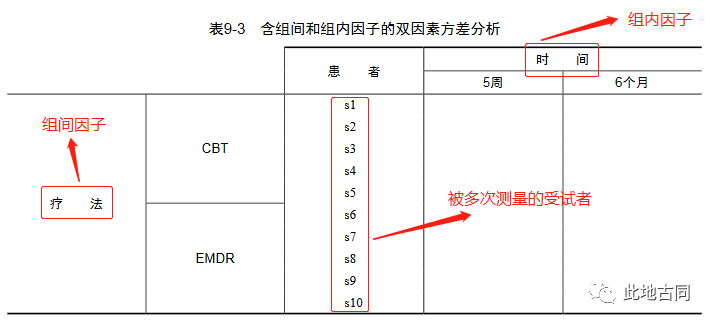2

数据集

``````#查看数据集前几列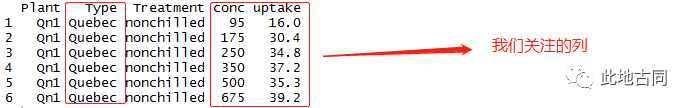``````#查看两个影响因子的列联表
table(CO2\$Type,CO2\$conc)``````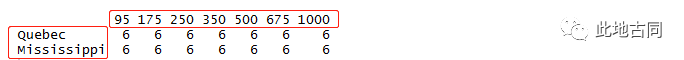3

检验假设条件

1.正态性检验

``````#加载程序包
library(car)
#正态性检验
qqPlot(lm(uptake ~ Type + conc,data = CO2),simulate = TRUE,main = 'Q-Q Plot',
labels=False,ylab = 'Value')``````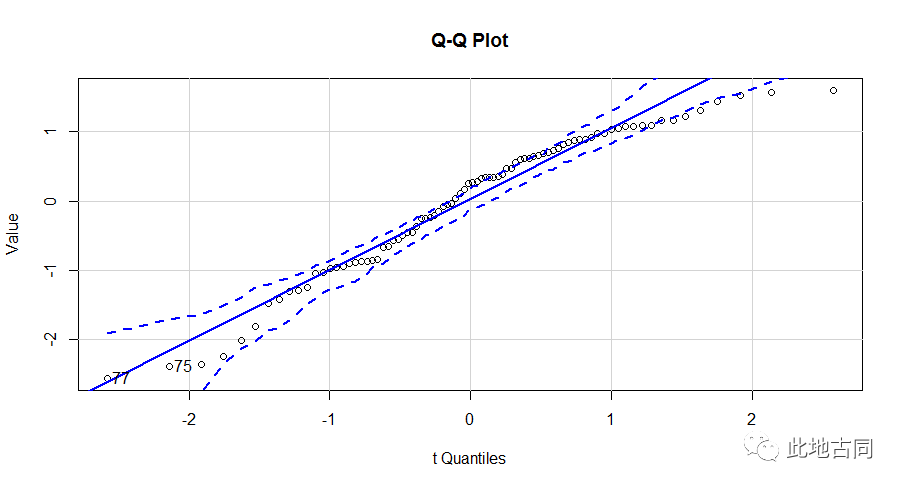2.方差齐性检验

``````#方差齐性检验
bartlett.test(uptake ~ Type,data = CO2)``````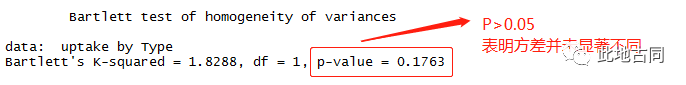4

描述性分析

``````#使数据集保持打开
attach(CO2)
#计算均值
aggregate(uptake,by=list(Type,conc),FUN=mean)``````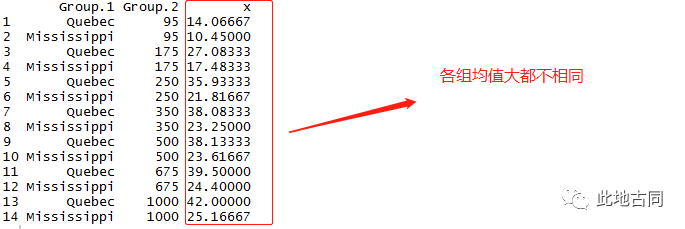``````#计算标准差
aggregate(uptake,by=list(Type,conc),FUN=sd)``````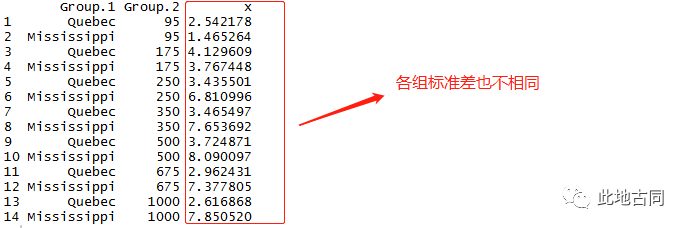5

重复性方差分析

``````#将conc转换为因子
CO2\$conc <- factor(CO2\$conc)
#提取出chilled处理列，控制变量
wlbl <- subset(CO2,Treatment == 'chilled')
#进行方差分析
fit <- aov(uptake ~ conc*Type + Error(Plant/(conc)),wlbl)
#查看结果
summary(fit)``````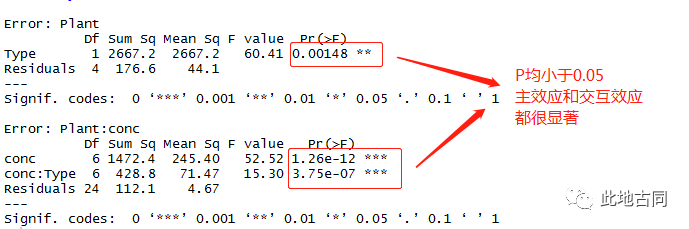<a>  二氧化碳吸收量与植物类型有关

<b>  二氧化碳吸收量与二氧化碳浓度有关

一图胜千言之绘图

``````#加载程序包
library('ggplot2')
#计算uptake均值并存储
data <- aggregate(uptake,by=list(Type,conc),FUN=mean)
#以上面的计算结果绘制均值变化曲线
ggplot(data = data,mapping = aes(x=Group.2,y=x,colour=Group.1)) +
#绘制点图
geom_point() +
#绘制折线图
geom_line() +
#更改X轴标题
xlab(label = 'conc') +
#更改Y轴标题
ylab(label = 'Mean of uptake') +
#设置背景背景
theme_bw() +
#设置XY轴标题的类型
theme(axis.title = element_text(family = 'serif',face = 'italic'))``````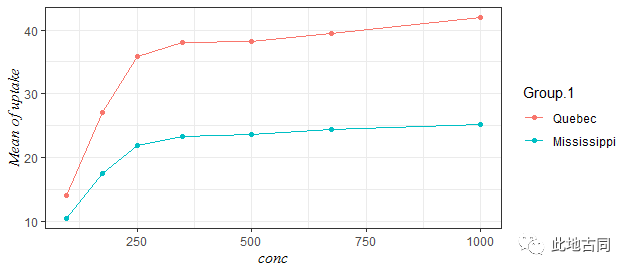``````#绘传入数据
ggplot(data = wlbl,aes(x=conc,y=uptake,colour=Type)) +
#绘制箱型图
geom_boxplot() +
#设置背景主题
theme_bw() +
#更改主题元素
theme(panel.background = element_blank(),
panel.grid = element_blank(),
axis.title = element_text(family = 'serif',face = 'italic'))``````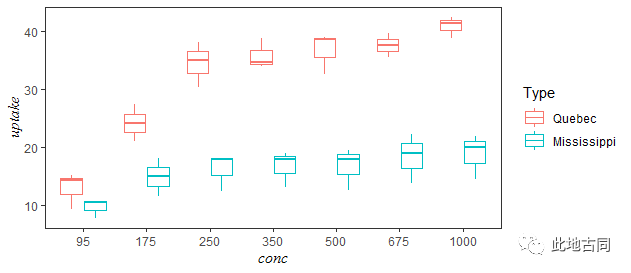你可能还想看